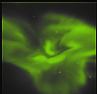Next: Atmospheric absorption Up: The Forward model - Previous: Voxel Pixel   Contents

# Pixel field-of-view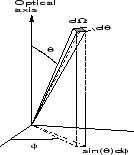In order to calculate the approximate intersection volume of the voxel in equation (5.3), the pixel field-of-view must be calculated.

Once the parameters of the optical transfer function are determined as outlined in chapter 4, the pixel field-of-view,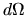, can be determined by standard calculus. With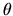as the angle relative to the optical axis of the camera, andas the azimuthal angle, the field-of-view is calculated as:(5.14)

for whichare the horizontal and vertical image coordinates and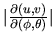is the absolute value of the determinant of the Jacobian of the optical transfer function, which, for the ALIS cameras, is given by equations (4.6 and 4.7).

copyright Björn Gustavsson 2000-10-24